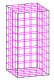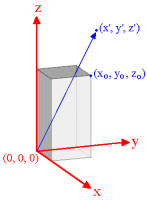CALCULATORS

### Magnetization CalculatorThe following calculator performs a volume integration of the magnetization to find the magnetic field at any chosen point (x’, y’, z’). The source of the field is a rectangular bar magnet with constant magnetization in the z direction. The magnet geometry is specified by the coordinates (xo, yo, zo) of the the corner opposite to the origin. The units for the length is irrelevant. The model is broken into “elements” by specifying the number of divisions in each direction.#### Bar Magnet Calculator Description

##### Demonstration
Number of Divisions Bz
x y z
1 1 2 0.057342936533257706
2 2 4 0.055563105597222495
4 4 8 0.05549498181873149
8 8 16 0.05549049917298043
16 16 32 0.05549021801106313
32 32 64 0.05549020042574602
64 64 128 0.05549019932647551
128 128 256 0.05549019925775747
256 256 512 0.05549019925342357

The default problem settings query the field at a height of 1 above the center of the magnet. The aspect ratio of the magnet is 1X1X2, so the minimum number of divisions with cubic element shape is 2. By repeatedly doubling the number of divisions in each direction and recalculating we can refine the solution and estimate how accurate the previous solution was. For example, there is a 3% decrease between steps 1&2 (0.0573 to 0.0558). This suggests that step 1 was accurate to within 3%

Following the trend it is clear that on average there is an increase in accuracy by a factor of about 20 every doubling. Note that doubling the number of divisions in each direction will increase the total number of elements, hence the total number of calculations, by a factor of 2x2x2=8. Depending on your computer you will probably notice the time taken becoming significant at approximately 50 divisions in each direction.

(For a more detailed description of ideal bar magnet benchmarking see the Bar Magnet Benchmarks.)

##### Method and Limitations

While the method used bears some similarities to our software’s solvers, it is setup as a fool-proof demonstration of a specific case, for calculations of an ideal constant magnetization material. It is not a real problem solver. Hence this page and/or the accompanying javascript is intended for benchmarking and educational or illustrative purposes.

In this calculator the magnet is broken up into rectangular elements, and the dipole moment of each element is calculated from its volume times the magnetization. The contribution of a selected point to the B field is then calculated by considering the element to be a magnetic dipole located at its center. Notice that in the demonstration case above it can be seen that for a distance of about 1 element length away the computation is accurate to within about 3%, for two element lengths away it is within about 0.1%. The computation will continue getting more accurate the higher the Distance:ElementSize ratio. (Note, one of our competitive advantages is the proprietory methods developed for performing integrals near elements and singularities.) The approximation works best for cubic elements, so it is worth considering the ratios of the side lengths when deciding how many divisions to use in each direction.

More Realistic Computations Using AMPERES with 24 elements

dB/dH Bz
1 0.055490202
1.01 0.05533903
1.05 0.054721256
1.1 0.053981921

The simple algorithm described above is foolproof, but impractical for large problems and only setup for rectangular bar magnets. It also disregards any surroundings for the bar magnet, and perhaps the biggest limitation in comparing with real bar magnets is the assumption of constant magnetization. For a real magnet there is a demagnetization of each part due to the presence of the other parts. The extent depends on the shape of the B-H curve for the material (which itself is history dependent due to hysteresis). The constant magnetization approximation means dB/dH=1. A real magnet will not generally be 1, however, for many magnets the slope is not much different. In this case the magnetization of the material is not uniform and the assumptions of this calculator break down. (Because of this, AMPERES begins by solving the boundary value problem.)

Comparing the calculations shown for AMPERES with those on the previous table demonstrates how much more efficient our software is than this simple calculator. The accuracy AMPERES achieves with 24 elements is not achieved until 32x32x64=65,536 elements with the calculator. This should provide confidence in the accuracy of AMPERES answer for the non-ideal cases as well. As shown above, the calculator becomes inaccurate in the percentage range for the given position with various nearly ideal magnets.

Using AMPERES at a height of 0.1 above the magnet

dB/dH Bz
1 0.39434642
1.01 0.39258272
1.05 0.38570575
1.1 0.37748873

Closer to the magnet the relative change due to non-ideal magnets is larger than farther away. The reader can verify with the calculator above the AMPERES is still yielding the correct answer to several decimal places.

The approximately 6% error showing up in this table may not seem that bad to some readers, especially those who are only familiar with pure Finite Element Method computations rather than the Boundary Element Method computations we use by default. Anybody wishing to make their own variations for particular problems can examine the source for this page and download the file. Those with real simulation problems requiring accurate solutions can use this calculator to make up some of the benchmarks for any software they try to pass. Note that for ideal material you should work in Gauss/Oersted units and set Br & Hc to the same numerical value in the material description in the software. We expect you’ll find no other product can achieve the same accuracy in as little time as AMPERES.